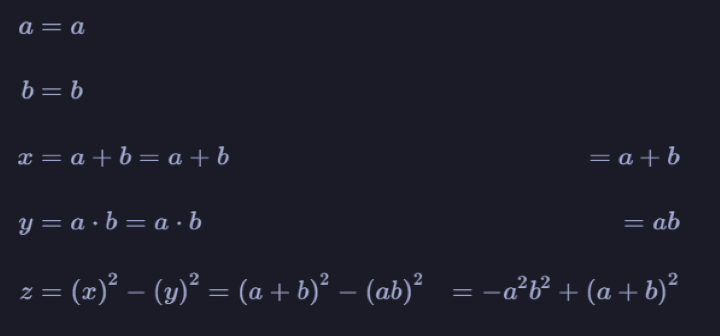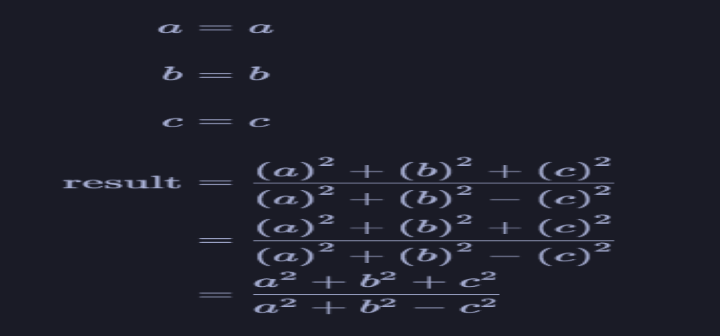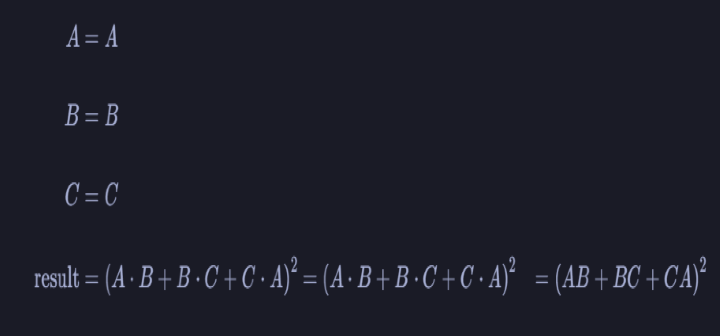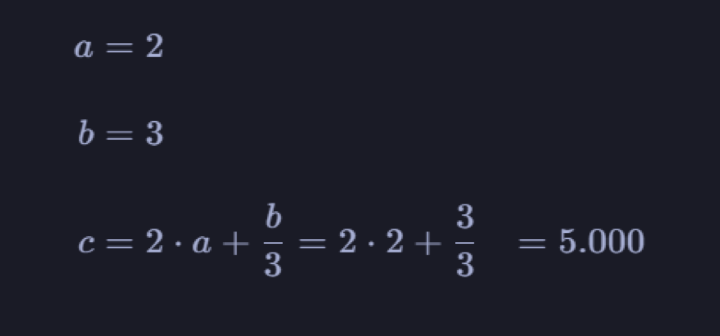# HandCalcs Module Python

HandCalcs is a Python module for automatic Latex report generation from Python code with minimal coding effort. HandCalcs uses Python's symbolic math library to track calculation history and translate it into LaTeX code. It can be used to automatically generate mathematical equations in LaTeX format. This module is a must-have tool for any Python developer who frequently uses LaTeX for technical documentation.

## Syntax

To install HandCalcs, use the following command in the terminal or command prompt −

!pip install handcalcs


To use HandCalcs in your Python code, first import the handcalcs.render function. This function is used to create a LaTeX report from the Python code. To begin, create a cell with the Python code you want to use, along with any relevant LaTeX commands, and then run the handcalcs.render function.

## Algorithm

• Define your mathematical expression using standard Python syntax. You can use variables, functions, and mathematical operators such as addition, subtraction, multiplication, and division.

• Wrap your mathematical expression inside a Handcalcs cell by enclosing it in triple braces (i.e., {{{}}}).

• Use the Handcalcs render function to create a report that includes the hand-written-style calculation. For example, you can use the code "handcalcs_rendering('report_name.tex', 'report_template.tex')" to create a report named "report_name" using the LaTeX template file named "report_template".

• Compile the LaTeX report using a LaTeX compiler such as TeX Live or MiKTeX to generate the final PDF report.

• Review and refine the report as needed, making adjustments to the code and template until the desired report format is achieved.

## Code

import handcalcs.render


Run the following in the next Jupyter Cell.

## Example 1

%%render
a = Symbol('a')
b = Symbol('b')

x = a + b
y = a * b
z = x**2 - y**2


## Explanation

This example shows how symbolic math calculations on variables can be carried out using HandCalcs. The two variables a and b are defined, and the variables x and y are created through addition and multiplication processes. The third variable, z, which is the outcome of a more difficult algebraic equation, is then calculated using x and y.

## Output## Example 2

%%render
a = Symbol('a')
b = Symbol('b')
c = Symbol('c')

result= (a**2 + b**2 + c**2)/(a**2 + b**2 - c**2)


## Explanation

The three variables a, b, and c are defined here as well and are used to compute an outcome. The final step involves using HandCalcs to show the expression in LaTeX style.

## Output## Example 3

%%render
A = MatrixSymbol('A', 2, 2)
B = MatrixSymbol('B', 2, 2)
C = MatrixSymbol('C', 2, 2)

result = (A*B + B*C + C*A)**2


## Explanation

This exercise illustrates how to manipulate matrices symbolically using HandCalcs. A, B, and C, three matrix factors that are defined, are used to compute the outcome, and the final equation is shown in LaTeX style.

## Output## Example 4

%%render
a = 2
b = 3
c = 2*a + b/3


## Explanation

This illustration shows how to use HandCalcs to carry out fundamental numerical arithmetic tasks. It sets the three variables a, b, and c and applies mathematical operations to determine the resultant number c

## Output## Conclusion

We explored the HandCalcs module in Python and its various features. We learned how to install HandCalcs, import the handcalcs.render function, and define HandCalc functions using the @handcalc decorator. We then created a sample script that showcases some of the main functions of HandCalcs. Overall, HandCalcs is a powerful tool for anyone who frequently uses LaTeX for technical documentation. With HandCalcs, you can automate the generation of mathematical equations and simplify the process of creating technical reports and documentation.

Updated on: 09-May-2023

225 Views